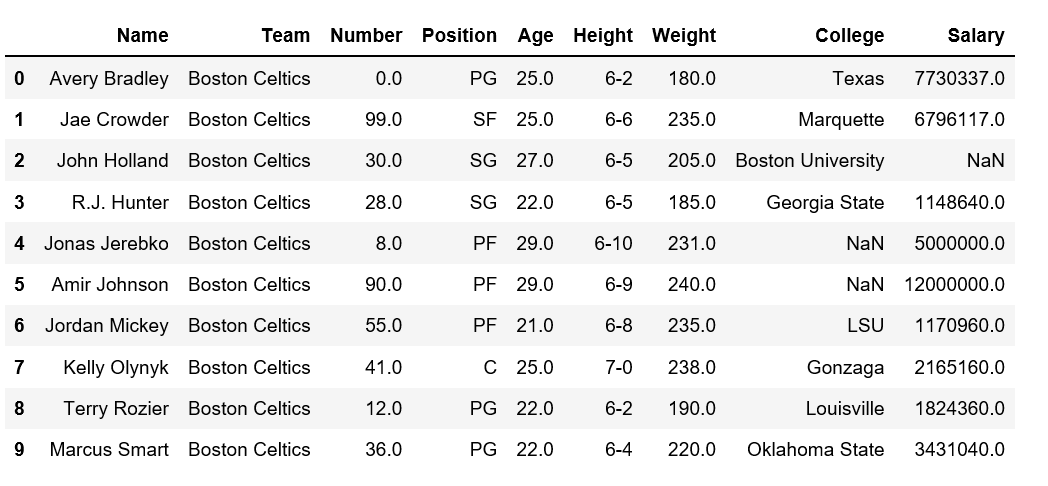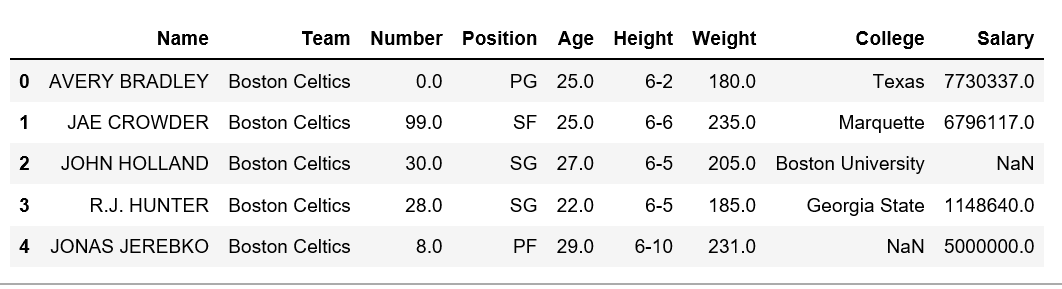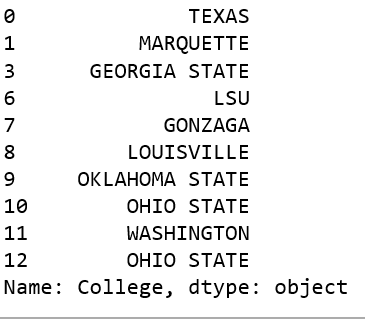# Apply uppercase to a column in Pandas dataframe

• Last Updated : 06 Dec, 2018

Analyzing a real world data is some what difficult because we need to take various things into consideration. Apart from getting the useful data from large datasets, keeping data in required format is also very important.

One might encounter a situation where we need to uppercase each letter in any specific column in given dataframe. Let’s see how can we Apply uppercase to a column in Pandas dataframe.

Let’s create a dataframe using `nba.csv`.

 `# Import pandas package ``import` `pandas as pd ``   ` `# making data frame ``data ``=` `pd.read_csv(``"https://media.geeksforgeeks.org/wp-content/uploads/nba.csv"``) ``   ` `# calling head() method  ``# storing in new variable ``data_top ``=` `data.head(``10``) ``   ` `# display ``data_top`

Output:There are certain methods we can change/modify the case of column in Pandas dataframe. Let’s see how can we apply uppercase to a column in Pandas dataframe using `upper()` method.

Method #1:

 `# Import pandas package ``import` `pandas as pd ``   ` `# making data frame ``data ``=` `pd.read_csv(``"https://media.geeksforgeeks.org/wp-content/uploads/nba.csv"``) ``     ` `# display ``data[``'Name'``] ``=` `data[``'Name'``].``str``.upper()`` ` `data.head()`

Output:Method #2: Using lambda with `upper()` method

 `# Import pandas package ``import` `pandas as pd ``   ` `# making data frame ``data ``=` `pd.read_csv(``"https://media.geeksforgeeks.org/wp-content/uploads/nba.csv"``) `` ` ` ` `# removing null values to avoid errors  ``data.dropna(inplace ``=` `True``)  `` ` `# Applying upper() method on 'College' column``data[``'College'``].``apply``(``lambda` `x: x.upper()).head(``10``)`

Output:My Personal Notes arrow_drop_up